# Back-Propagation is very simple. Who made it Complicated ?

## Learning Outcome: You will be able to build your own Neural Network on a Paper.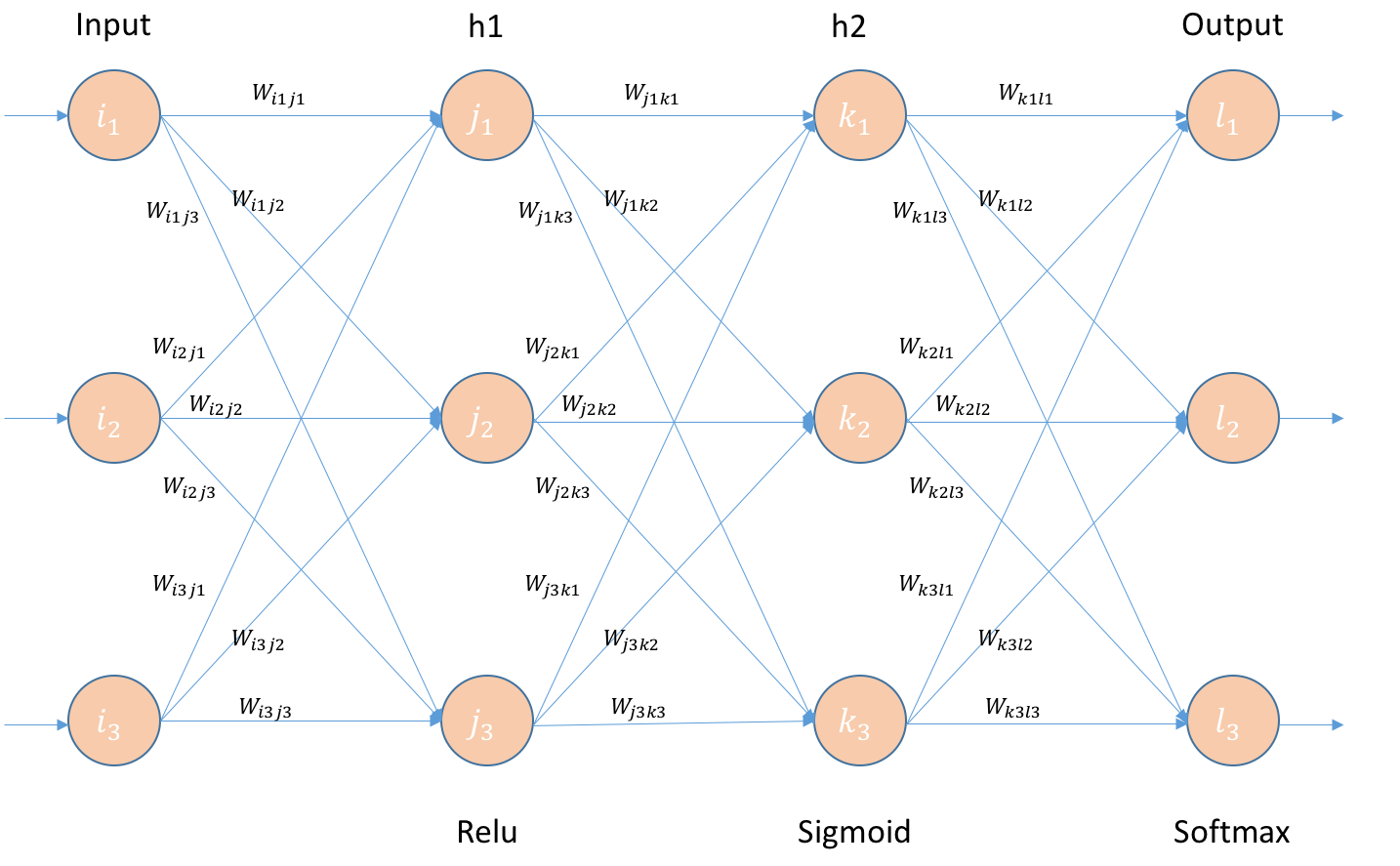Feed Forward Neural Network

Almost 6 months back when I first wanted to try my hands on Neural network, I scratched my head for a long time on how Back-Propagation works. When I talk to peers around my circle, I see a lot of people facing this problem. Most people consider it as a black-box and use libraries like Keras, TensorFlow and PyTorch which provide automatic differentiation. Though it is not necessary to write your own code on how to compute gradients and backprop errors, having knowledge on it helps you in understanding a few concepts like Vanishing Gradients, Saturation of Neurons and reasons for random initialization of weights

Andrej Karapathy wrote a blog-post on it and I found it useful.

• Build a small neural network as defined in the architecture below.
• Initialize the weights and bias randomly.
• Fix the input and output.
• Forward pass the inputs. calculate the cost.
• compute the gradients and errors.
• Backprop and adjust the weights and bias accordingly
• Build a Feed Forward neural network with 2 hidden layers. All the layers will have 3 Neurons each.
• 1st and 2nd hidden layer will have Relu and sigmoid respectively as activation functions. Final layer will have Softmax.
• Error is calculated using cross-entropy.

I have taken inputs, weights and bias randomly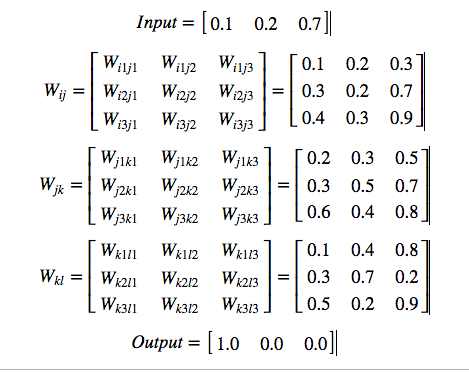Initializing the network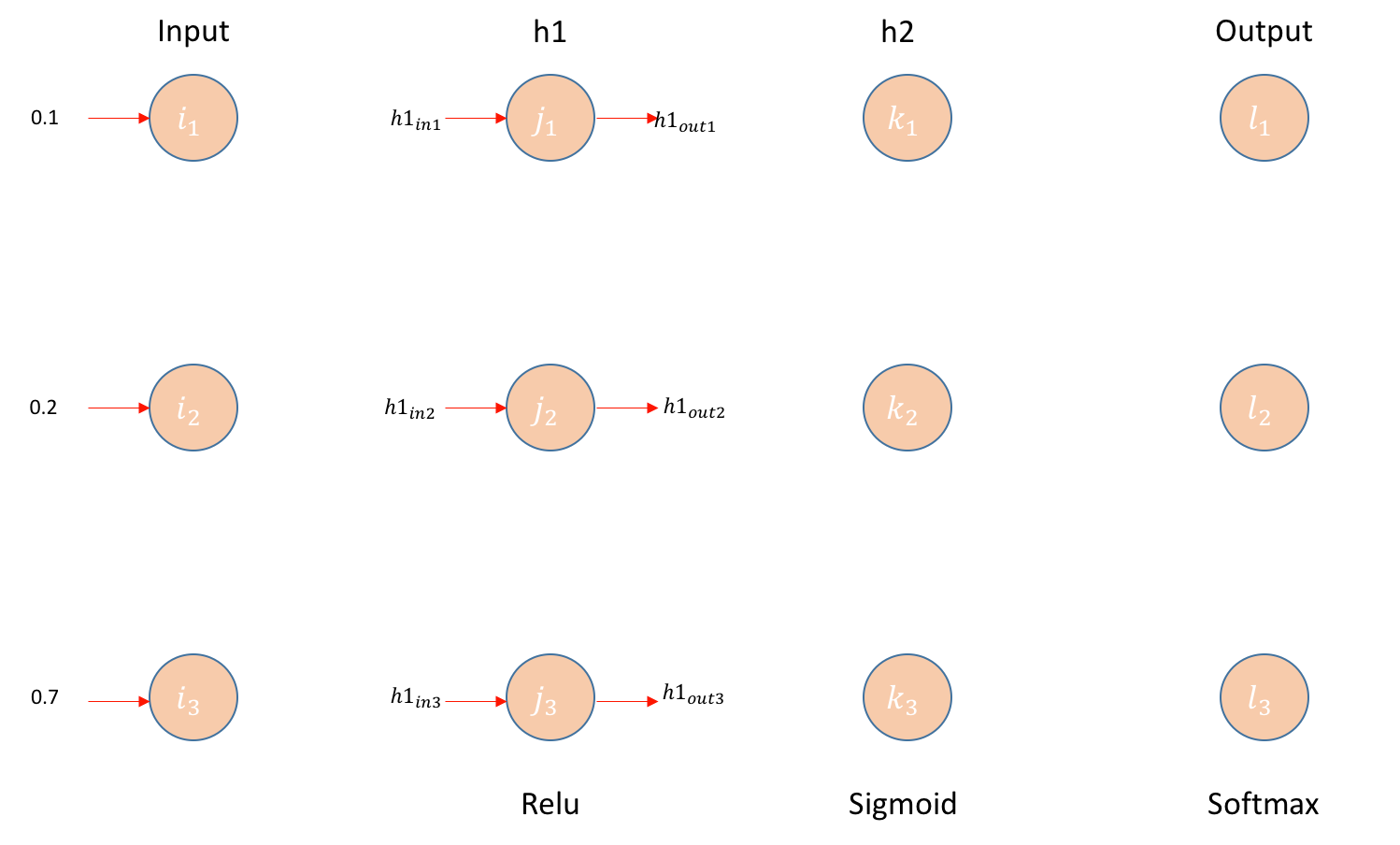Neural Network Layer-1

Matrix Operation: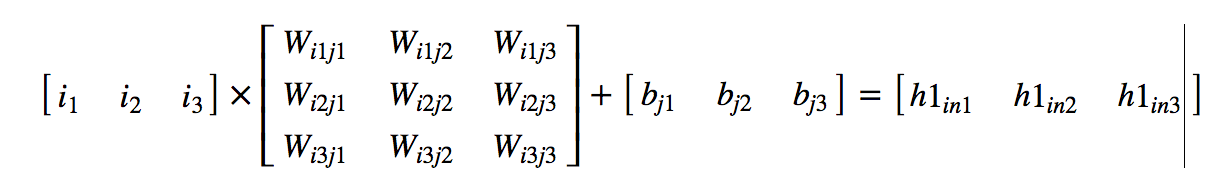Layer-1 Matrix Operation

Relu operation: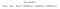Layer-1 Relu Operation

Example: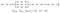Layer-1 ExampleLayer-2 Neural Network

Matrix operation: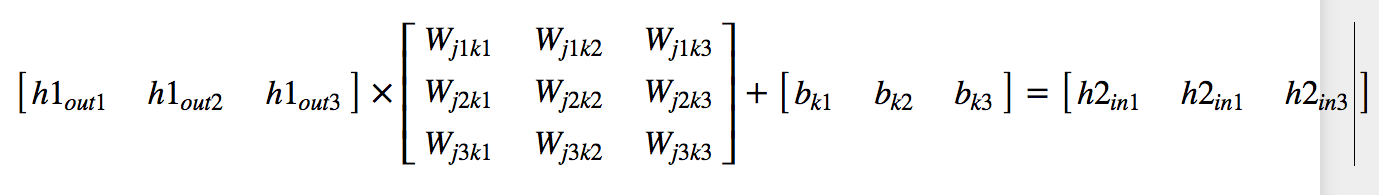Layer-2 Matrix Operation

Sigmoid operation: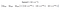Sigmoid Operation

Example: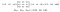Layer-2 Example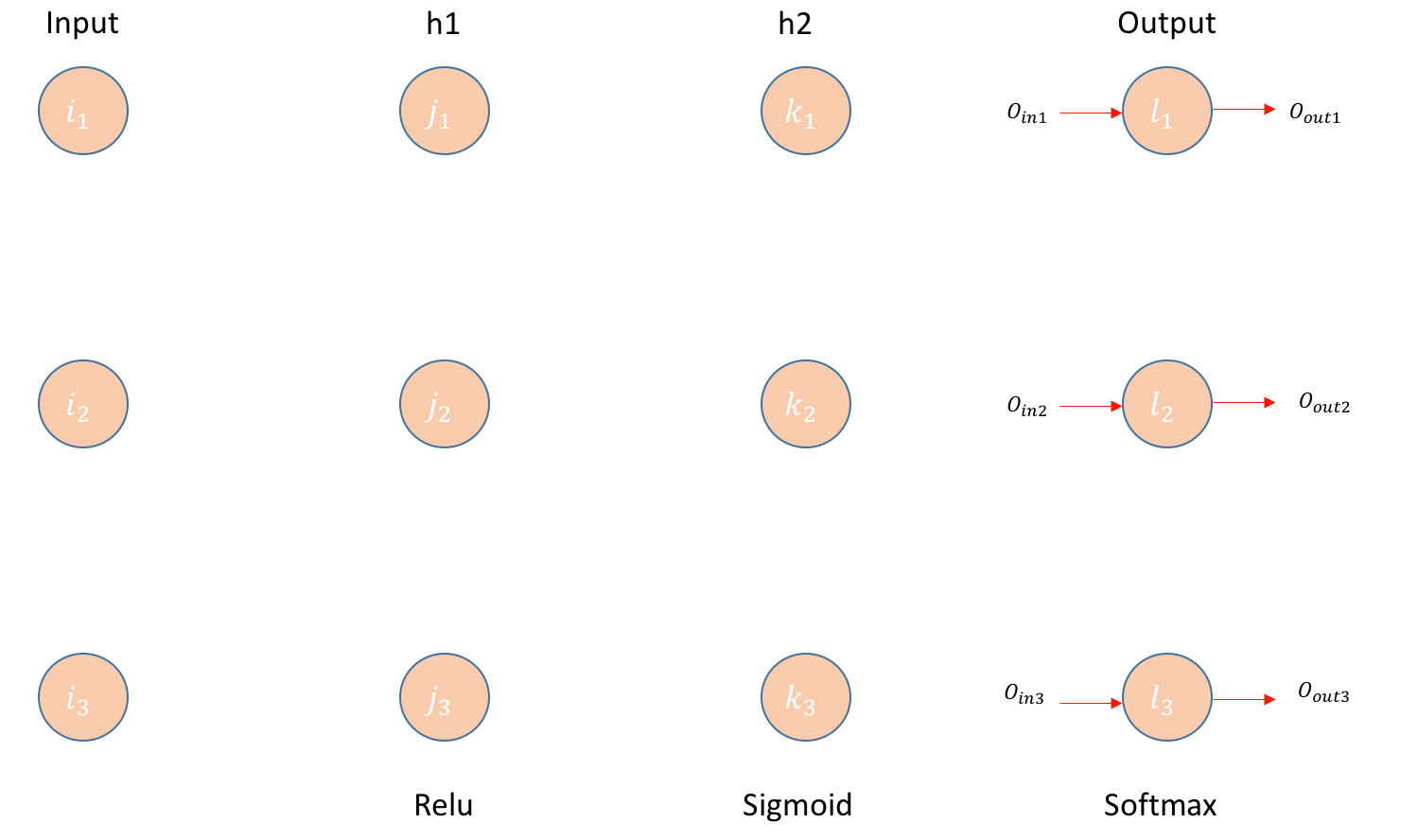Layer-3 Neural Network

Matrix operation: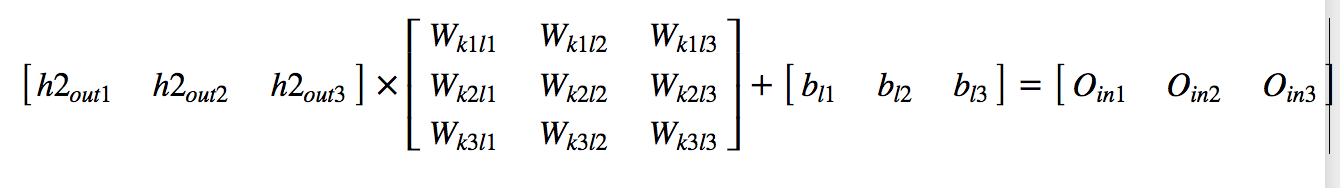Layer-3 Matrix Operation

Softmax operation: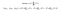Softmax formula

Example: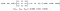Layer-3 Output Example

Edit1: As Jmuth pointed out in the comments, the output from softmax would be [0.19858, 0.28559, 0.51583] instead of [0.26980, 0.32235, 0.40784]. I have done a/sum(a) while the correct answer would be exp(a)/sum(exp(a)) . Please adjust your calculations from here on using these values. Thank you.

Analysis:

• The Actual Output should be [1.0, 0.0, 0.0] but we got [0.2698, 0.3223, 0.4078].
• To calculate error lets use cross-entropy

Cross-Entropy: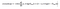Cross-Entropy Formula

Example:Cross-Entropy calculation

We are done with forward pass. Now let us see backward pass

# Important Derivatives: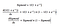Derivative of Sigmoid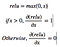Derivative of Relu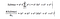Derivative of Softmax

# BackPropagating the error — (Hidden Layer2 — Output Layer) Weights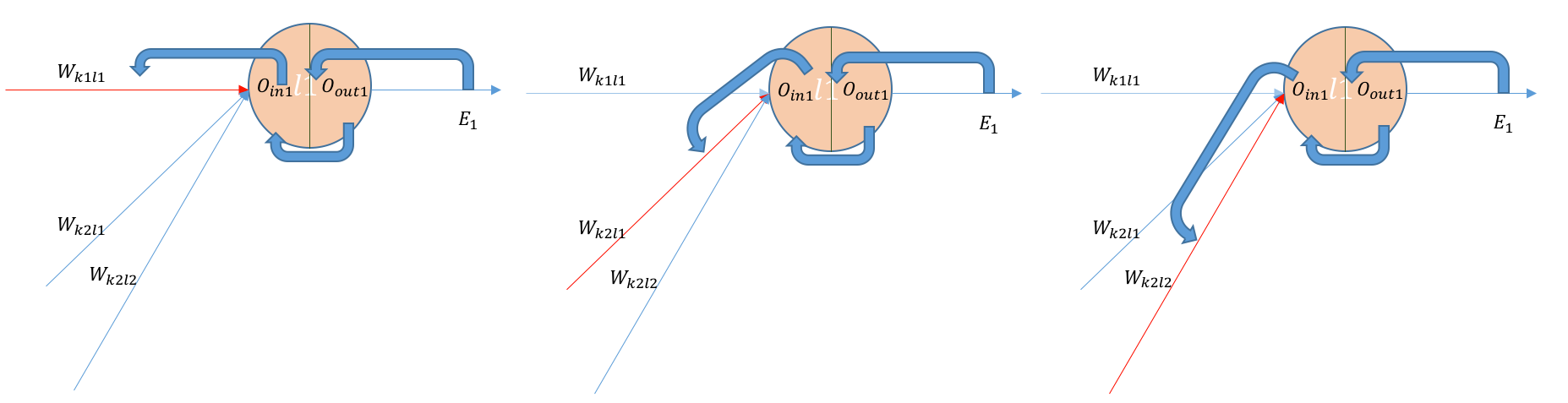Backpropagating Layer-3 weights

Let us calculate a few derivatives upfront so these become handy and we can reuse them whenever necessary. Here are we are using only one example (batch_size=1), if there are more examples, We just need to average everything.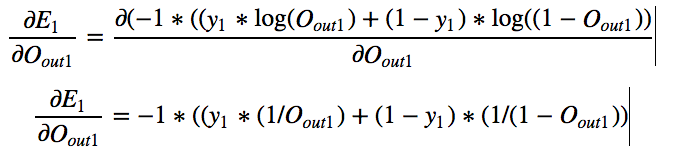Example: Derivative of Cross-Entropy

By symmetry we can calculate other derivatives also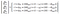Matrix of cross-entropy derivatives wrt output

In our example,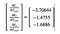values of derivative of cross-entropy wrt output.

Next let us calculate the derivative of each output with respect to their input.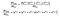Example: Derivative of softmax wrt output layer input

By symmetry we can calculate other derivatives also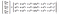Matrix of Derivative of softmax wrt output layer input.

In our example,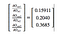values of derivative of softmax wrt output layer input .

For each input to neuron let us calculate the derivative with respect to each weight. Now let us look at the final derivative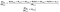Example: Derivative of input to output layer wrt weight

By symmetry we can calculate other derivatives also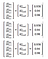values of derivative of input to output layer wrt weights.

Finally Let us calculate the change in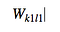Weight from k1 to l1 neuron

Which will be simply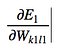Derivative of error wrt weight

Using Chain Rule: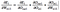Chain rule breakdown of Error derivative

By symmetry: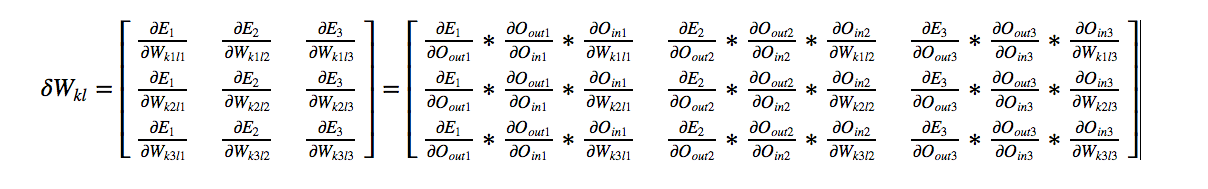Matrix Form of all derivatives in layer-3

All of the above values are calculated before. We just need to substitute the results.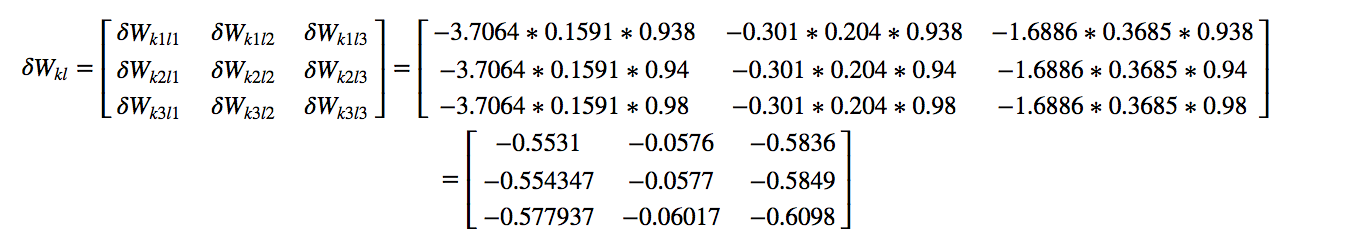Example: Calculation of all the values

Considering a learning rate of 0.01 we get our final weight matrix as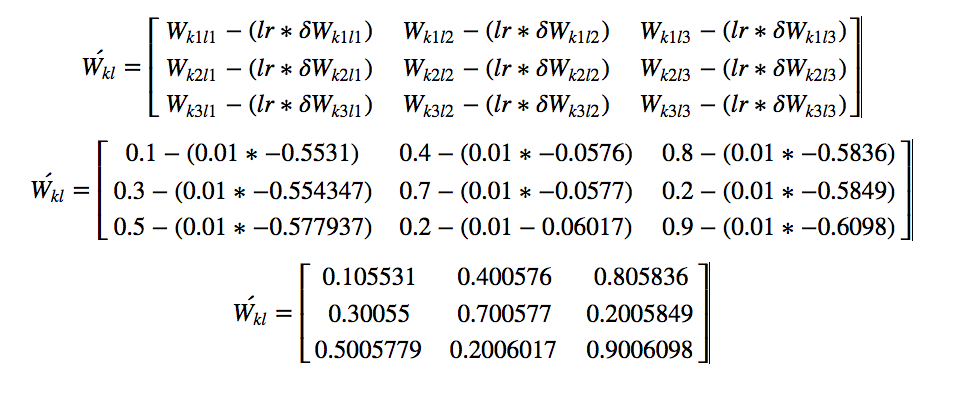Modified weights of kl neurons after backprop

So, We have calculated new weight matrix for W_{kl}. Now let us move to the next layer:

# BackPropagating the error — (Hidden Layer1 — Hidden Layer 2) Weights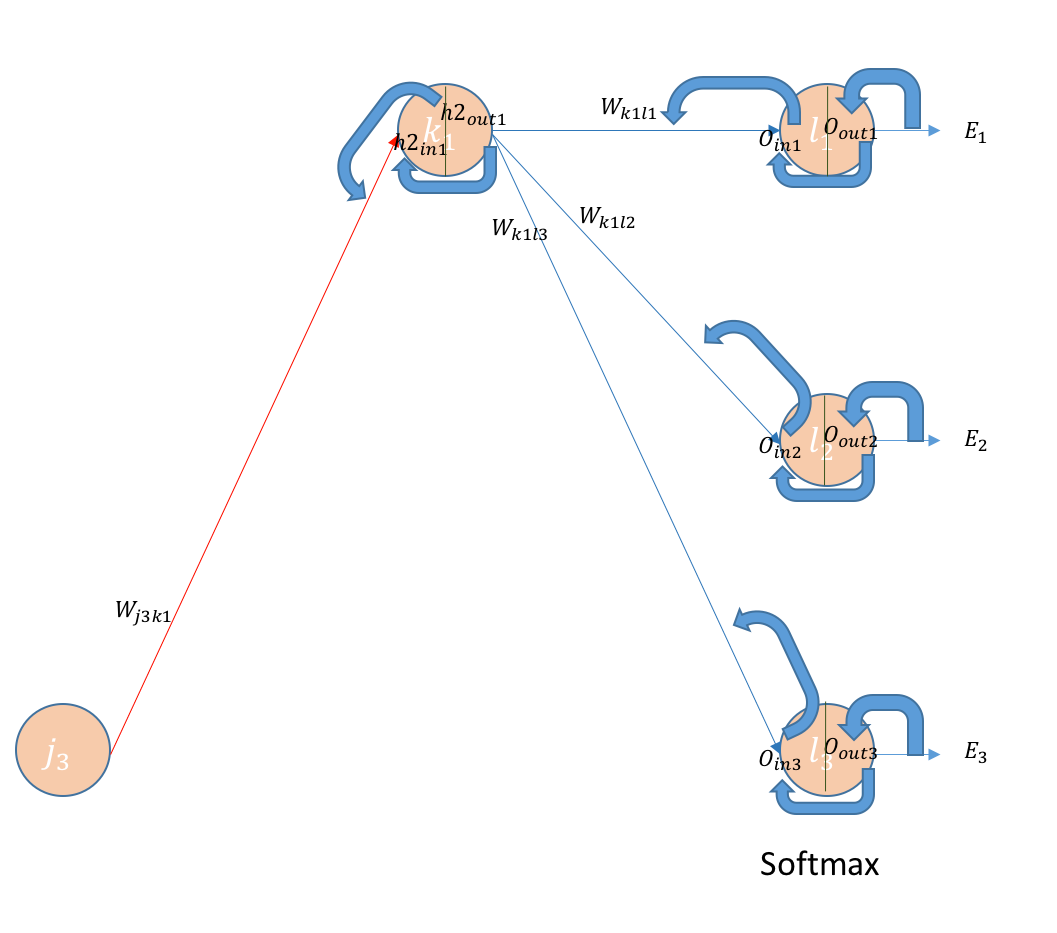Backpropagating errors to 2nd layer

Let us calculate a few handy derivatives before we actually calculate the error derivatives wrt weights in this layer.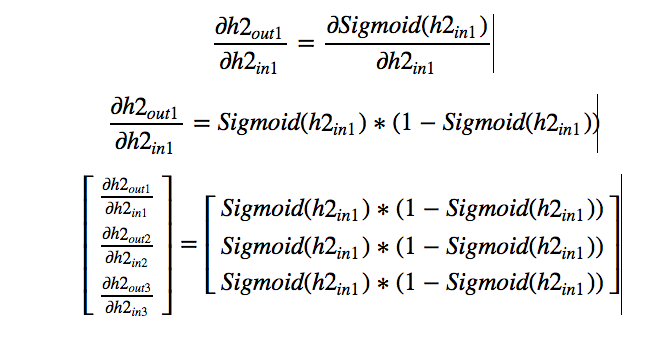Example: Derivative of sigmoid output wrt layer 2 input

In our example: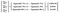Values of derivative of output of layer-2 wrt input of layer1

For each input to neuron let us calculate the derivative with respect to each weight. Now let us look at the final derivative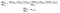Derivative of layer 2 input wrt weight

By symmetry we can calculate: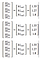Values of derivative layer 2 input wrt of weight

Now we will calculate the derivative of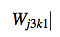weight from j3 to k1

which will be simply.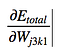Derivative of Error wrt weight j3-k1

Using chain rule,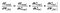chain rule of derivative of error wrt weight

By symmetry we get the final matrix as,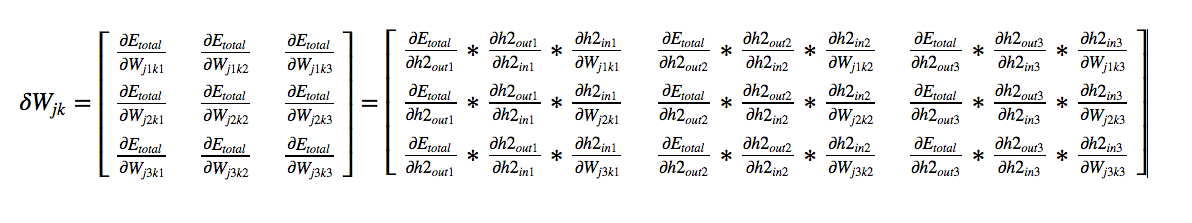final matrix of derivatives of weights_{jk}

We have already calculated the 2nd and 3rd term in each matrix. We need to check on the 1st term. If we see the matrix, the first term is common in all the columns. So there are only three values. Let us look into one value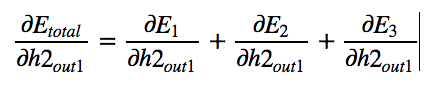Breakdown of error.

Lets see what each individual term boils down too.breakdown of each error derivative

by symmetry we get the final matrix as,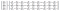Derivative of error wrt output of hidden layer 2

Again the first two values are already calculated by us when dealing with derivatives of W_{kl}. We just need to calculate the third one, Which is the derivative of input to each output layer wrt output of hidden layer-2. It is nothing but the corresponding weight which connects both the layers.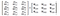Derivative of input of output layer wrt hidden layer -2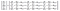Final Matrix of derivative of total error wrt output of hidden layer-2

All Values are calculated before we just need to impute the corresponding values for our example.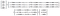calculations using an example

Let us look at the final matrix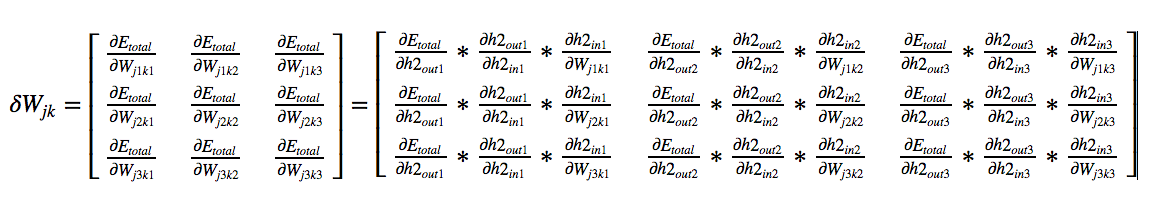Our final matrix of derivatives of Weights connecting hidden layer-1 and hidden layer-2

In our example,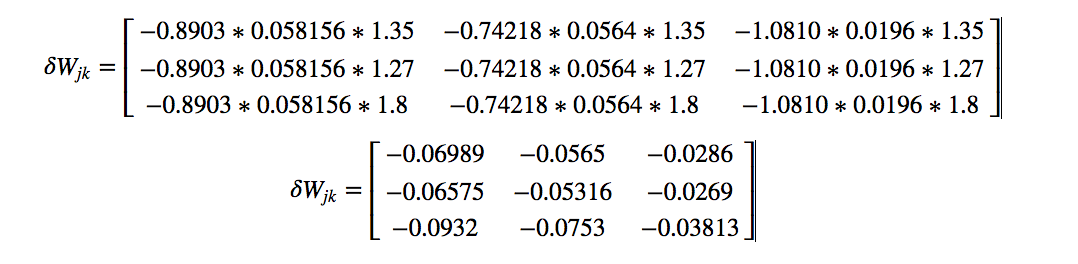Calculations from our examples

Consider a learning rate (lr) of 0.01 We get our final Weight matrix as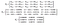Final modified matrix of W_{jk}

So, We have calculated new weight matrix for W_{jk}. Now let us move to the next layer:

# BackPropagating the error — (Input Layer — Hidden Layer 1) Weights.

Edit:1 the following calculations from here are wrong. I took only wj1k1 and ignored wj1k2 and wj1k3. This was pointed by an user in comments. I would like someone to edit the jupyter notebook attached at the end. Please refer to some other implementations if u still didn’t understand back-prop here.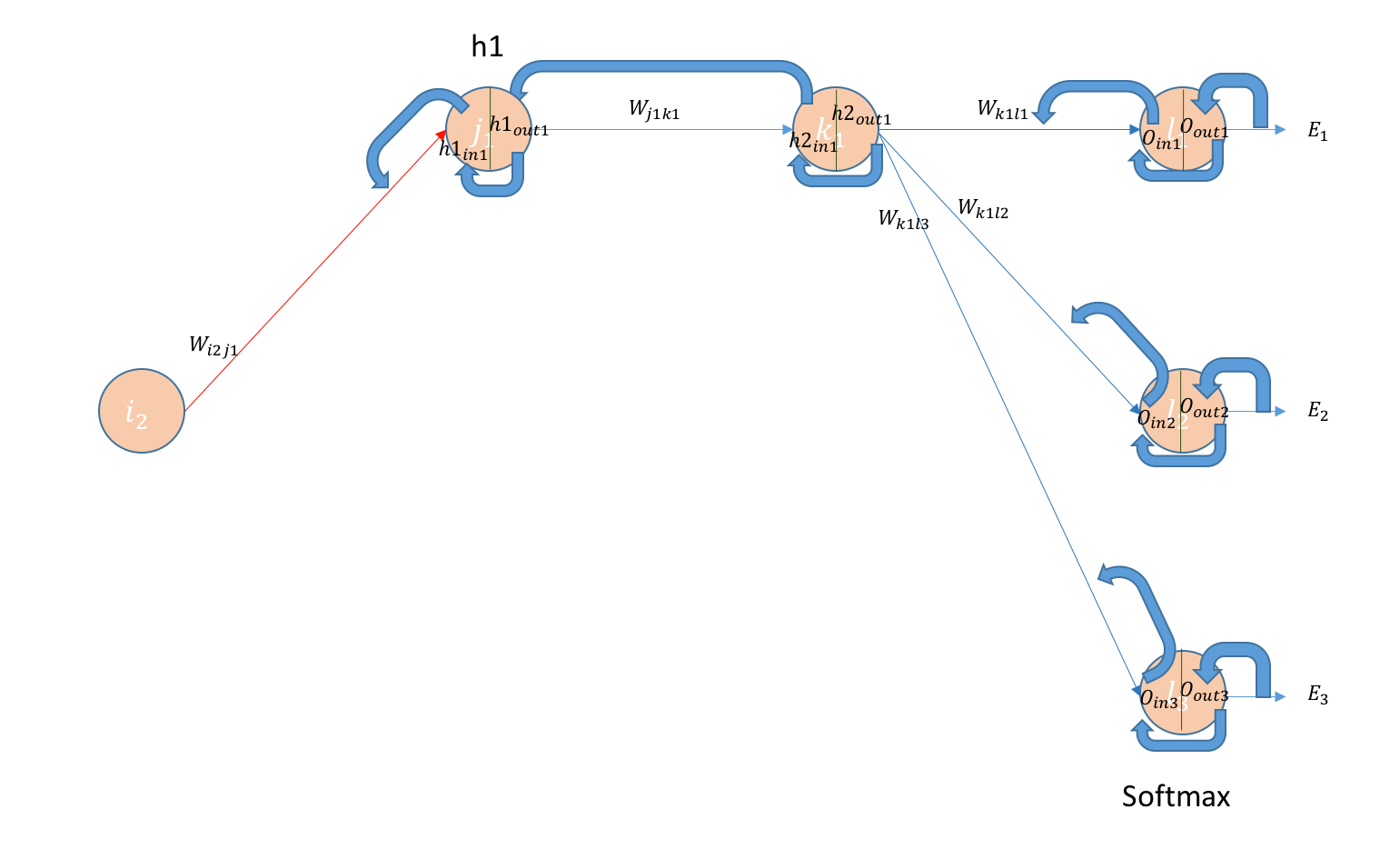Backpropagating errors to 1st layer

Let us calculate a few handy derivatives before we actually calculate the error derivatives wrt weights in this layer.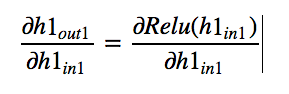Derivative of hidden layer 1 output wrt to its input

We already know the derivative of relu (We have seen it at the beginning of the post). Since all inputs are positive, We will get output as 1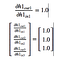Calculations

For each input to neuron let us calculate the derivative with respect to each weight. Now let us look at the final derivative.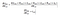Derivative of input to hidden layer wrt to weights

By symmetry we can write,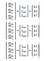Final derivative calculations

Now we will calculate the change in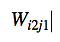Weight connecting i2 neuron to j1

and generalize it to all variables. This will be simply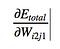derivative of error wrt to weight

Using chain rule,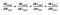chain rule for calculating error

By symmetry,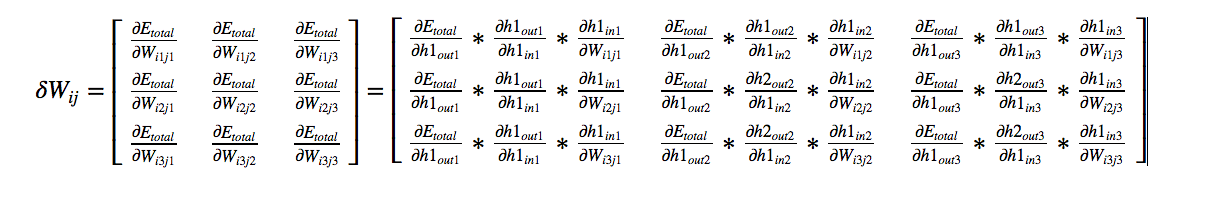Final matrix using symmertry

We know the 2nd and 3rd derivatives in each cell in the above matrix. Let us look at how to get to derivative of 1st term in each cell.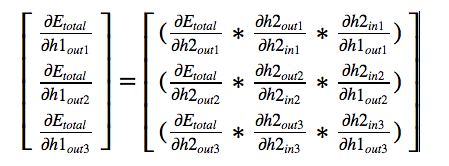Watching for the first term

We have calculated all the values previously except the last one in each cell, which is a simple derivative of linear terms.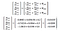calculations in our example

In our example,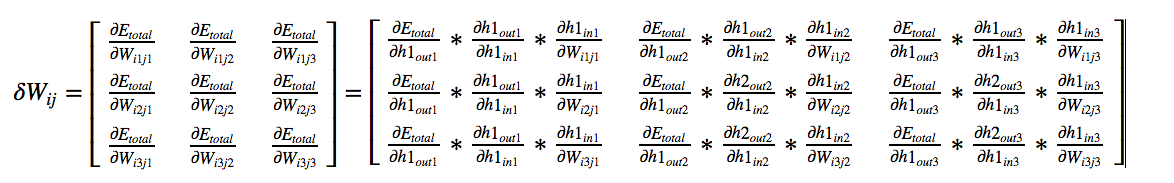Final matrix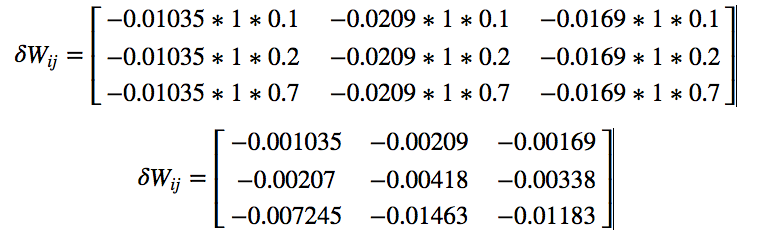calculations in our example

Consider a learning rate (lr) of 0.01 We get our final weight matrix as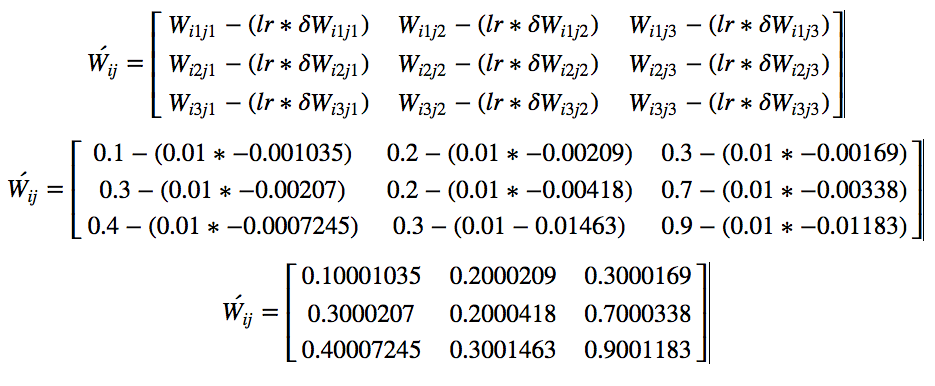using learning rate we get final matrix

# Important Notes:

• I have completely eliminated bias when differentiating. Do you know why ?
• Backprop of bias should be straightforward. Try on your own.
• I have taken only one example. What will happen if we take batch of examples?
• Though I have not mentioned directly about vanishing gradients. Do you see why it occurs?
• What would happen if all the weights are the same number instead of random ?

# Code available on Github :

I have hand calculated everything. Let me know your feedback. If you like it, please recommend and share it. Thank you.

Senior Data Scientist @FractalAI

Senior Data Scientist @FractalAI